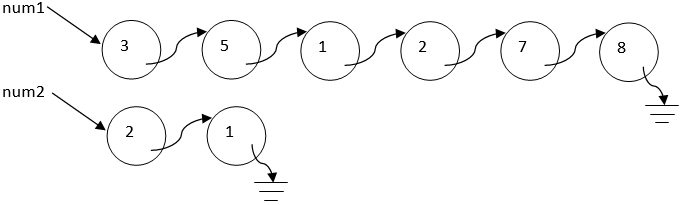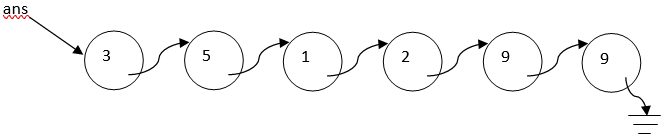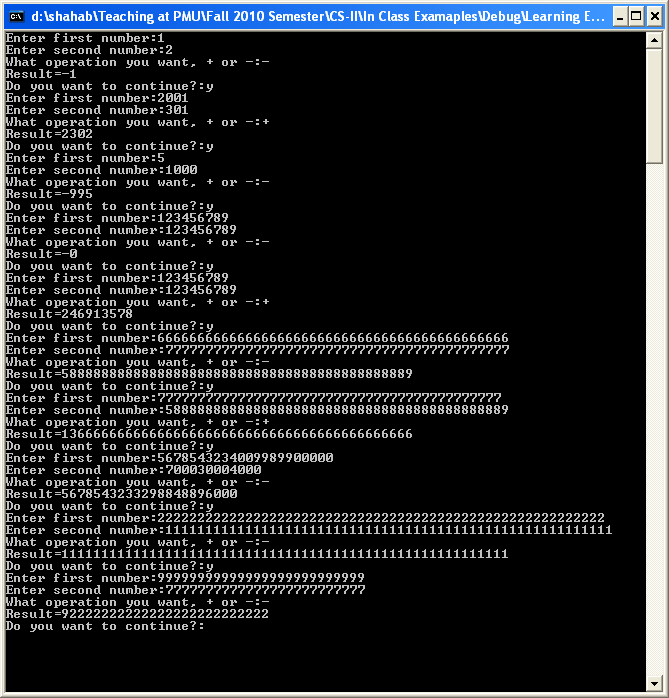Q:

# Write a program for a Calculator that can do three operations using java linkedlist

Write a program for a Calculator that can do three operations, +, –, and x. This calculator should be able to add, subtract, and multiply two numbers of any size. The following is the sequence of steps that your program should follow:

1. Ask user to enter the first number.
2. Your program should get this number from the user.
3. Ask user to enter the second number.
4. Your program should get this number from the user.
5. Ask user whether the user wants addition, subtraction, or multiplication. User will enter +, -, *.
6. Your program should perform the operation whatever user entered above.

Finally, your program should show the result in the output screen.

Now, in order to implement this calculator program, you need to remember the following:

1.  Take the first number from the user and store that number in a linked list. Let’s say the first linkedlist has head named ‘num1’.
2. Take the second number from the user and store it in another linked list. Let’s say the second linkedlist has head named ‘num2’.
3.  Remember that the user may enter a number of any size. Your program should keep on taking the input number until user hits the Enter key. As soon as the user hits Enter key, your program should know that the user is done entering the first number. Now your program should repeat this process and take second number from the user.
4.  If the user enters the following number: 45683450976535456 and hits Enter, it means your code should create the first linked list of 17 nodes because there are 17 digits in the abovementioned number. Similarly your program will create another linked list of n nodes where n is the number of digits in the second number
5. Let’s say, the user enters 351278 as the first number and 21 as the second number. So after taking both numbers from the user, this will be the situation:6. Now if user entered +, then all you need to do is to add the above two linked list and create aSample Output: